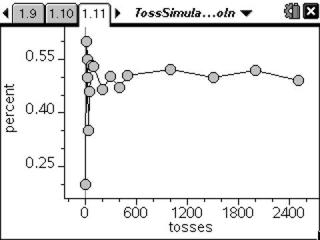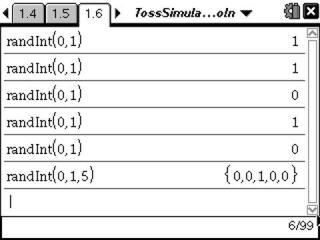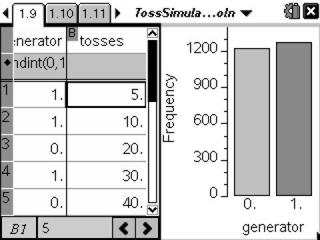# Activities

••• ##### Subject Area

• Math: Calculus: Applications of the Derivative
• Math: Statistics: Probability and Random Variables

• ##### Author9-12

20 Minutes

• ##### Device
• TI-Nspire™ Navigator™
• TI-Nspire™
• TI-Nspire™ CAS
• ##### Software

TI-Nspire™
TI-Nspire™ CAS

2.0

## To Toss or To Simulate?#### Activity Overview

Students use the RandInt() command to simulate the tossing of a coin and the rolling of a die. They will collect data for several trials and use the information to investigate the Law of Large Numbers.

#### Key Steps

•Students are introduced to using the Random Integer command to simulate an experiment. First, they will explore the difference between entering the command randint(0,1) five times and entering randint(0,1,5).

•Then, students will use the RandInt command in a spreadsheet to simulate the tossing of a coin for various number of trials. The bar graph on the right side of the screen will help them count the number of heads for trial.

•After all the data has been collected, students will calculate the percent of heads and create a scatter plot of percent of heads versus number of tosses. They can graph the theoretical probability as a horizontal line for comparison.